Select Page

## Our World is Inside Out

This is the most important post of them all. It not only ties them all together but will take us to another level entirely. Numbers are not what we think they are.  We could not be more wrong. Our thinking has been far too limited.

There are complex and abstract numbers, but we are referring to even the simplest single digit numbers. Numbers make up and describe our entire world. If we are in a simulation, even a Divine one, they are our world.  Some people like numbers, some are afraid of them. However, none of us actually understands them. We have been taught to view numbers in a passive sense, as a descriptive factor, such as 12 oranges doing nothing more than counting how many oranges are on the table.  What if numbers played a more active role in our world. What if 12 was the motive or the operational factor and the oranges were just the objects to help us visualize the concept of 12. For anyone that has not been following this series from its inception, you can find the entire work here.

### Positive and Negative Space

The negative space in the Magical Cube of 42 was determined to be a volume of 22,005 cubic units. What specific cubic units they are is irrelevant, as the space is virtual and just a projection from a higher dimension where our units of measure are meaningless. That was based on the sum of the 27 Hebrew letter values, positive space, being 4995, whose four digits sum to 27, but which came first, positive or the complimentary negative space? Yet the numbers themselves are not meaningless. For example, for whatever reason the natural logarithm of 22,005, or LN (22005), is 9.9990… or precisely 1/1000 less than a perfect 10. The value 1/1000 makes perfect sense to true Kabbalists and readers of this series given that the volume of this Magical Cube of 27 Letters operates in Binah hyperspace, a space based on the concept of 1000, or 103, and thus the Essential Cube’s total volume is (27 x 1000).

Have you ever asked why there are 10 Commandments; or 10 Plagues; 10 Patriarchs and Matriarch of collective value 2481; 10 primordial component letters; or 10 sefirot (dimensions)? Why 10?

### Abraham and Aaron

We know that the gematria of all 5 Book names in the Torah is 2480 and that of the two last Books, Bamidbar is 248 and Devarim is 256, matching the names Abraham and Aaron, 248 and 256 respectively.  We also know how both numbers connect to the E8 Lattice and how they connect in assorted ways throughout the (248 x 42) Torah matrix. What we have not discussed until now is that the natural logarithm of 12, or LN (12), or put another way, Loge (12), is 2.48490665 and that the natural logarithm of 13, the very next integer, or LN (13), or Loge (13), is 2.564949357, with a difference of .08004270 between them.  This cannot be coincidental. Natural logs are not just another way to generate one number from another; they are used in exponential growth, similar to what we saw in the exponential curve of the Alef-bet. The two letters that correspond to the ordinal values 12 and 13 are Lamed (ל) and Mem (מ), whose combined numerical value is 70, as in the 70 years of King David and all the other 70 year periods redundantly repeated throughout the Divine Calendar of history. Moreover, (2 + 4 + 8) + (2 + 5 + 6) = 27.

Maybe, we do not really understand what numbers are.

Spiritually, both Abraham and Aaron represent “love.” The Kabbalists have always told us that gematria and the numbers convey the deepest meanings of the Torah to us, the 70th face of the Torah, and we have learned throughout this series that numbers can convey meanings to us no matter where we find them. Yet, those are still passive conveyances. Why are the numbers 248 and 256 next to each other Biblically and through the natural logarithms? What do the number 12 and 13 have to do with it?

The answer to those questions lies beyond our comprehension. We can only do simple computations like (12 x 13) = 156, the numerical value of Joseph and Zion, and fit them in chronologically to the Divine Calendar, where we see that Joseph was born in 2200 HC, 252 (6 x 42) years after Abraham in 1948 HC, and 248 years before the Exodus from Egypt. Joseph passed away 55 (2 x 27.5) years before Aaron was born in 2365 HC.

### It Always Goes Back to the Cube.

In the Magical Cube of 42, there are 27 numbers from 127, arranged is a very particular order, with the number 14 in the center. Each of the 27 numbers matches a different ordinal value for each of the 27 Hebrew letters, these total 378 for the 27 ordinal values and 4995 for the 27 actual letter values. Altogether, (27 + 378 + 4995) = 5400, which works out to (200 x 27). Is this why there are 54 portions in the Torah? Or is this the reason: the sum of the digits in (27 + 378 + 4995) = 54?

In an equation, what comes first the solution or the components? What about in the higher dimensions? Must the digits sum up specifically before the components or answer is chosen? That cannot be done on our level; one should not have anything to do with the other. It is like saying 12 cherries and 12 apples = 24 oranges and by the way their volume is equal too.

### 72 Triplets

Do you recall early on in this series that the odd-even split of the Alef-bet was off from the Phi(φ) split by a value of 54, and that the sum of the square roots of the 72 Triplets as Triplets and letters was 1000.054? Now we know why.

There are 27 sub-cubes within the Magic Cube of 42 and since each of those sub-cubes has 8 corners, there are a total of 216 corners within the Magic Cube of 42, as in the 216 Letters in the 72 Triplets.

There are 27 sub-cubes within the Magic Cube of 42 and since each of those sub-cubes has 26 elements (8 corners, 12 edges, 6 faces) there are a total of 702 elements within the Magic Cube of 42, as in the numerical value of Shabbat (702) that exists on a higher dimension and constitutes the 7th millennium.

All numbers connect. It is a matter of untangling their web.

### Untangling the Cube

Besides the 27 ordinal values, the total value of the 27 numbers is naturally also 378, which works out to (14 x 27), thus highlighting the central cube and central placement of the number 14.  All 3 components of (27 + 378 + 4995) = 5400 are each multiples of 27.

The value 378 built into the equation is exactly 7% of 5400.  It does not just work out that way; it was designed that way, and not by us. It was built into the Cube and the 27 letters of the Alef-bet.

The number 378 is the value of “Echad Ushmo Echad (אחד־ושמו־אחד)” or “His Name is One,” whose final letters spell David (דיד) of numerical value 14.

The number 27 is exactly .5% of 5400. It does not just work out that way; it was designed that way, and not by us. It was built into the Cube and the 27 letters of the Alef-bet.

### 3 Values; 3 Levels; 3 Dimensions

There are 3 tiers to this equation. The numbers and/or values 27, 378, 4995, all are equivalent to each other in their own dimensions.  All 3 numbers are expressions of the same concept. The number 27 exists in the realm of Binah; the number 378 exists in Zeir Anpin; and the number 4995 for the value of the same 27 Hebrew letters exists in Malchut. All 3 are “27” in the dimension of Binah. All are One (1) in the realm of Chochma. Therefore 27 is One(1).

No letter exists separately from the whole. No number exists separately from One(1).

We look at numbers as all existing in the same plane, linearly, much as we view time.  This is extremely limiting and in the case of time, lethally limiting. The universe is not constricted by these limitations and is not linear. Geometry is non-Euclidean, and as we have seen, all these key numbers that keep coming up in every aspect of mathematics and throughout the structure of the Torah, and within the mathematical and physical constants themselves, all interconnect and intersect continually, fluidly. They even intersect with the key markers on the linear timeline that we have carved out.

The 27 Primordial forces or pressure mediations within the inertial fields are One (1) and undifferentiated in Chochma, the 9th dimension. When they reach malchut they are represented by 4995, but they are still 378 all together, and 27 individually and 1 as an undivided, undifferentiated whole.  Yet they never actually separated. They are all still altogether 5400 and all still One(1) at once.

This is a difficult concept to get our heads around, yet it is critical to escape the limitations and boundaries of this world.  There is a relationship between the numbers in these different levels but that is not what connects them. A simple mathematical ratio between them would only reveal their relationship in the level of Malchut.  What we call mathematics in the upper dimensions looks very different. For example, in our realm distance is measured in set, fixed units; in the upper dimensions distance is determined by similarity of form and is flexible.

Gematria, by revealing some of those similarities, helps us perceive the origins of the effects of the primal forces on our world. Detecting intersections and patterns between the numbers highlights those similarities and origins as well.  Neither is meant to lead to a comprehension of the higher dimensions, but to the awakening that they exist. That awakening will lead to enlightenment.

### A Place in Pi

The gematria of the word “Torah” is 611, and the 611th digit in Pi is 1. It is immediately preceded by …127… The sum of all the digits in Pi through digit #611 is 2700.  The numbers 1 and 27 have everything to do with the Torah, whose first verse has a numerical value of 2701. Numbers are active operators in our world, not just passive markers. Most people have not even realized that numbers are even markers. There are numerous significant multi-dimensional sequences within Pi, as will be illustrated below. It should be kept in mind that when the sum of the digits through a specific sequence is significant on its own and/or through its interconnectivity it is telling us that every single digit (number) prior to it was purposely chosen to be there. The only way for that to have happened was at the moment of Creation.

By the way, the constants operate on the level of Binah, meaning that when we reach into them, we are tapping into the limitless Sea of Binah. While we can find notable sequences anywhere within the endless digits of Pi, we have only to look within the first 1000 as 1000 is the level of Binah.

### Mod 9

Another pattern in this equation (27 + 378 + 4995) = 5400 is revealed by analyzing the sum of the integers in each number. This equation thus translates to (9 + 18 + 27) = 9.  Another aspect of math is called modular arithmetic. It is a system of arithmetic for integers, where, like a clock  numbers “wrap around” when reaching a certain value, called the modulus. In the case of a clock, it is mod 12. In the case of the above equation it is logical to apply mod 9, where a number is equivalent to “0” whenever it wraps around to “9.”  Thus all the components in this equation are “0” in Mod 9 both in their original form and also in their secondary reduced form: (0 + 0 + 0) mod 9 = 0 mod 9.

By dividing both sides of (9 + 18 + 27) = 9 by 9, the reduced (translated) equation transcribes to (1 + 2 + 3) = 1, illustrating how these 3 levels equal One(1).

This may also be incorporated into the first line of the 42-Letter Name matrix (אבגיתץ), which begins with 1 and ends with 27, and moreover begins 1,2,3,1 in small gematria, as in (1 + 2 + 3) = 1.

### Understanding Why

The total ordinal value of the 27 Hebrew letters (378) in the above equation helped give us 5400. The total value of the 27 numbers is naturally also 378. If we add that 378 to 5400, we get 5778. Is this the reason that 5778 HC was the chosen year, and a span of 5778 years was the chosen time frame? Since the dawn of Creation, that year has always been written into Pi, Phi, base 10 mathematics, and the Torah, but is this the reason it was so indelibly engraved?

The year 5778 is part of a narrow cosmic window, a multi-millennial opportunity that for the most part is being wasted thanks to a clever opponent. Someone on the path to enlightenment would easily recognize the number 5778 as a marker, and specifically a marker in time.  Someone more enlightened would realize 5778 was not just a marker, but the source code, a driving force unto itself. Someone actually enlightened would understand why.  The realm of Binah translates to Understanding. Only at the level of Binah can we have true Understanding, everything else is a process to get there.

Given the relationship in the equation (27 + 378 + 4995) = 5400 to the number 9, and that the sum of the digits in (27 + 378 + 4995) = 54, how interesting that the 3rd of the triplet digit string 5778 in Pi is found at digit # 954.  To understand numbers, we must think multidimensionally. They do not exist in a vacuum and have little to no meaning statically.  It is like a line of computer code: while it may be significant, without all the other lines of code it cannot do anything.

Since the equation (27 + 378 + 4995) + 378 = 5778 breaks down to 27(1 + 14 + 185 + 14) = (27 x 214), we see that this Essential Cube of Creation equation at its core is 27 times the numerical value of ruach (רוח), 214, spirit, as in the second verse of the Torah, “G-d’s spirit moved on the water’s surface.”  Not coincidentally, this verse has 14 words, as in 378/27 = 14, which takes us to the center of the Cube(14), much like David (14) was at the center of the 5778-year time span.  The more we understand about a number the more we can interact with it. The more we interact with them the greater our connection to the upper dimensions. Rav Abulafia, of blessed memory, advised us that the highest understanding of the Torah was through the numbers.  The number 5778, whether on a calendar or on a license plate is meaningless without a conscious interaction. It is like having a cell number, you have to use it for it to mean anything.  The Hebrew letters are just letters on paper without the primordial forces they connect too, likewise the numbers that help us interface with them.

When we now look at the number 5778, we can now see the Spirit (רוח) of the Creator in it, like a soul in a body. As that Spirit (27 x 214) fills all the 27 sub cubes of a 33 cube with 214 (רוח) in each, we can now picture the number 5778 as a specific cube. Indeed, we can picture it as the Essential Cube of Creation.

## Accessing the Essential Cube of Creation

The Spirit (ruach) of the Creator is in the Cube, in 5778. It breaches all 3 levels: Malchut, Zeir Anpin, and Binah. It is in every aspect of the Essential Cube of Creation. It makes the Cube, One(1).  And 1/5778 = .0001730…, as in the small gematria of the 42-Letter Name of G-d. Our understanding thus deepens, and our consciousness expands. We know how to use the 42-Letter Name. It was the first gift of the Bible given to us. Since 5778 x 1/5778 = 5778/5778 = One(1), we can see how connecting the 42-Letter Name with the year (number) 5778 and the window of opportunity it brings us, can bring us closer to  One(1) and the One. Moreover, we see how connecting the 42-Letter Name with the year (number) 5778 can open up the power of the Essential Cube of Creation and help us access the Binah hyperspace.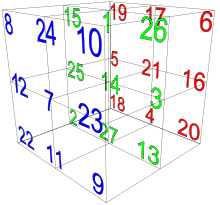Rav Brandwein, of blessed memory, said that it is through the understanding of the 42-Letter Name that we will bring about the Geula (final redemption). Remember, the Essential Cube of Creation is the Magical Cube of 42 since it was designed so that each of the 27 rows and columns would total 42.  Furthermore, because of this design, each of the 27 letters of the Hebrew Alef-bet are connected to the number 42 and the 42-Letter Name. Perhaps this is why the Arizal, may his memory be for a blessing, said that no prayer elevates without the help of the 42-Letter Name.  This is our access to all points of the Essential Cube of Creation, our gateway to all points beyond.

### The Square Root of Two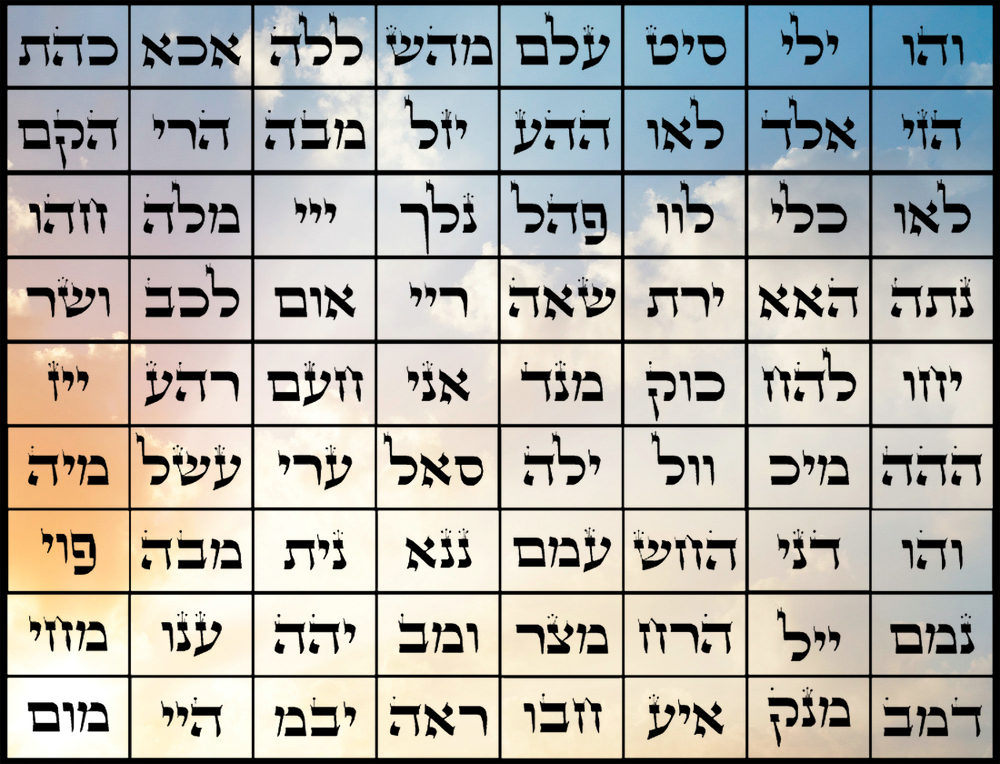Mathematics works different on different levels (dimensions). We have previously illustrated that taking the square root of the product of specific equations, like the values in the P/S cipher, yields its essence. That is how mathematics in our world can show us a glimpse of the higher nature of those numbers.  In the level of Zeir Anpin, when we sum up specific numbers , take their square roots and sum those up, as with the two components of the 72 Triplets that worked out to 720.0 and 280.0 for a total of 1000.054, we get an even higher glimpse into the source of those numbers.  We can apply that within the Essential Cube of Creation.

The Essential Cube of Creation is designed so that the value 14, which as an ordinal number is at the level of Zeir Anpin, can be readily combined with every other letter in the Cube. Utilizing all 27 letters, that combination totals 378, which works out to be (14 x 27). When combined with the 27 Hebrew letters, which are on the level of Malchut, it adds 378, and, as said previously, combined with the level of Zeir Anpin it also adds a total of 378. The central (14) can combine with the level of Malchut as in the example of Resh (200) + 14 = 214, ruach (רוח). Or it can combine with the level of Zeir Anpin, as when it combines with itself: (14 + 14) = 28, as in Koach (כח), power, and as in the 28 letters of the Torah’s first verse that has the Malchut value 2701, or (27 + 1) = 28.

When all 27 ordinal values of Zeir Anpin are combined with their corresponding Zeir Anpin (14) value, the total is (378 + 378) = 756, whose square root is 27.5, the measure of the sacred cubit.  When are the square roots of all 27 combined values (15, 16…41) are summed up, the result is 100 times the square root of two(2): 141.4227 or (100 x 1.414227) vs the precise square root of 2,  1.414214, off by only 0.0000134275….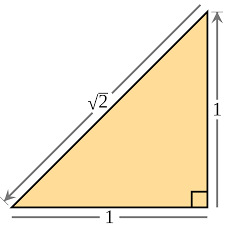And we thought the square root of 2 was just a number used in geometry, notably with the squaring of circles.  Only when we expand our consciousness can we see the connections, began to grasp the vastness and effect of multiple dimensions acting simultaneously.  The Zeir Anpin – Zeir Anpin equations within the Cube above give us new connection points or portals (√2 and 14, 14, 42, and 27), which are all also components of the equation, indicating a circling or self-referencing nature to the multi-dimensional system. The √2 itself gives us 14, 14, 42, and 214, ruach (רוח) which we know is at the heart and essence of the Cube. Perhaps this is not so surprising since the √2 represents the diagonal of any square of unit side length One(1) and thus of each of the 6 sides of a similar cube, or each of the 27 planes, but we did not use geometry to get to this value (√2). Mathematics works differently in the higher dimensions. Distance is measured by similarity of form. Their difference (0.0000134275….) gives us 13, 42, and 275 translated as Echad/One(1), 42, and the sacred cubit again.

Without tracing the connections, numbers are just limitations. Without the right consciousness tracing the connections is limited. Without the desire for elevation, attaining the right consciousness is futile.

# He and his Name are One

The concept of “He and his Name are One” is part and parcel incorporated into the negative and positive space within the Cube. As perceived in the higher dimensions the letters and the space that defines them are One and the same. Take a blank sheet of white paper. Every point on this sheet, no matter how small, holographically contains the whole paper. Now trace out the Name of G-d, any one of them. What do you see? The white inside the Name is the same as the white outside it.  The name was there all along.  By adding something (our ink), we think we have created something new, but in actuality we have taken something away, reduced or restricted the Light of the Creator.  This is what happens with each level of metaphysical descent to our world.  All the perceived existence around us, is just an extreme restriction of the light force of the Creator. This includes time and the linear line of death.  The light-force must be reduced so many times (iterations)—as we illustrated long ago, 22 times per level—for multiple levels for us to perceive our own existence. As beautiful as our world is, we are but seeing shadows of shadows of projections of projections.  Our world is so restrictive that we could be in a simulation and we would never know it. From the perspective of the Creator and the higher dimensions, it is all the same. A black sedan, a white coupe, a bicycle, no vehicle at all, life, death, all the same. Science will tell you the same. Cars, cows, life, death are all just 99.9999% empty space with a handful of pixie dust that we call quarks thrown in to interact with the fields of nothingness.

In the case of the Cube, the 22005 cubic units of emptiness (negative space) is exactly .815 of 27,000, or 81.5%.  We would have assumed that a perfect universe would have chosen 22,000 or (22 x 1000) to have worked out as a precise percentage of 27,000, given the relationship between the 22 Hebrew letters and the 5 final ones to make 27 in total. Nevertheless, that would be our limitation again. Whose is to say that in the higher dimension of the negative space that 22,000 is the same as it is in our limited world. The Creator specifically chose 22,005 out of 27,000 to portray that positive/negative relationship, the same way He chose 2701 to represent the Torah’s first verse and not 2700. In that specific case, He was illustrating to us that when a specific number equals the sum of all the integers through a particular number, like 73 in this case, representing the 9th sefira, Chochma, then that chosen number (2701) is operating on a higher plane or dimension. What is this like? It is like the 90,100 Yuds(י), Heis(ה), and Vavs(ו) in the Torah, given that 90,100 is the sum of the integers from 1 to 424, Moshiach Ben David.  Does this have anything to do with the nearest Star to our Sun being 4.24 Light years away? Probably, but it is not for us to find meaning in every connection, only to awaken to the reality that this universe was designed for us to awaken in.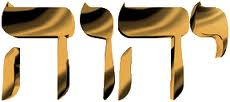Thus, we just learned another rule of higher dimensional mathematics. These rules do not show us what goes on mechanically in the higher dimensions; they just give us insights into them and insights in how to connect to them. Our goal, though, is not to unravel higher dimensional mathematics, but to expand our consciousness.

And just as 22005/27000 = .815, a nice short round rational number, so too is 4995/27000 = .185 a rational number, as is 4995/27 = 185, from the equations above. The number .185 is equivalent to 1/(5.400 +.005400 +. 000005400…), which means that the portion of the Malchut black fire letters to the whole (4995/27000) is equal to the One(1) divided by the whole(5400). Here is another example of higher dimensional mathematics at work, where we see the infinite echo of a number, like two mirrors facing one another. Again, these are representations of the numbers in the higher dimensions, not the mechanics of how the higher dimensions operate.  In our world 5.400 and 5400 and .000005400 are light years away from one another and you would much prefer \$5400 in your wallet than \$5.40, but in the upper realms, they are virtually alike.

The ratio 22000/27000 is not a rational number, and the next closest number to be rational is 21,978/27000; there is logically only one every 27 numbers. Can we understand numbers without comprehending the principles with which they were created? The letters and their defining space (4995 + 22005) are One; (4995 + 22005) = 1.

That (8 + 1 + 5) = (1 + 8 + 5) = 14 is just yet another example of how little we understand the interrelation of numbers, and how key the central cube of 14 is to the Essential Equation.

### 1 – 14 – 27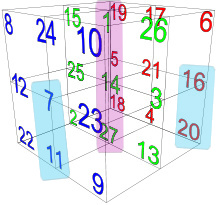This relationship of 11427 found throughout our discussion today is very clearly portrayed in the design of the Magical Cube of 42 since it represents the (11427) central vertical axis of the Cube and, as such, naturally sums to 42.

Also, within the cube we find two separate vertical pairs, the 711 (כז) pair that equals 27, and the 2016 (רע) pair that equals 270. Together the (711) and (2016) ordinal values = 54, as in twice 27 and as in the 54 portions in the Torah. Why these were built into the Cube, we may never know.

That key (11427) central vertical axis of the Cube also enables the gift-wrapping function of the Magical Cube of 42 to work in that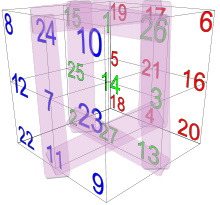each strap of the bow tied around the cube necessarily equals 112, as in the 112 Triplets that virtually orbit the Cube.

It should also be noted that the 112 Triplets contain 336 letters and (336 + 42) = 378 or (9 x 42). This is not higher mathematics, just an illustration that so much more is at work here than we realize.

There is a rarely used and deeper gematria cipher that is extremely revealing. We have illustrated how it can be used multiple times already, including to derive Pi from the Torah’s first verse. In this case, when we apply it to the key (11427) central vertical axis we get the Product of the components (1 x 14 x 27) divided by the Sum of the components (1 + 14 + 27) equals P/S = 378/42 = 9. Comprehension of the otherworldly nature of numbers is critical.

Furthermore, how is it, or why is it that (127/42) = 5778/ Phi(φ)?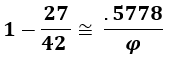### Pi, Phi and 5778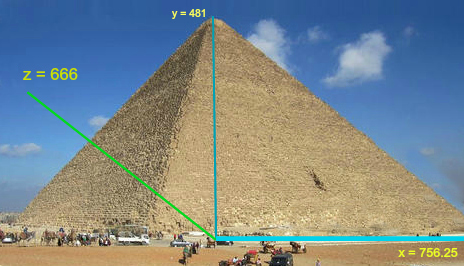The two values 378 in the equation (27 + 378 + 4995) + 378 = 5778 together equal 756, as in the total ordinal value, 756, of the 6 faces of the Magical Cube of 42; as in the base of the Pyramid (756’); and as in the spelled-out letter Nun(נון), equivalent to the ordinal value 14 at the center of the Cube.  Not only is 756 equal to (18 x 42), but Phi(φ)18 = 5778.000. Perhaps this is the reason the base of the Pyramid is 756’ and its height is 5775”.  The intersections between the numbers and disparate items in our world are there to awaken our understanding. The numbers are meaningless unless we see the intersections and the entanglements, and these too are meaningless unless we see the multiple dimensions simultaneously at work.  The sequence …57781857780… is found in Pi within the first 1000 digits and Phi(φ)18 = 5778.000. Pi (3.1415926…) and Phi (1.6180339887…) have very definite and significant effects upon our world. They convert lines into circles and rectangles into spirals. Our existence is predicated on their existence. Yet they actually exist in the hyperspace sea of Binah.  Why they intersect at this particular moment in hyperspace and how that translates into a window of time in our world is for us to fathom. As is also the role of the number 18.

### The Sacred Cubit

Was the relationship between 27 and One(1) the reason that the sacred cubit that the Pyramid and Holy Temples were based a on had to be 27.5 inches? Does it have to do with 27 being exactly .5% of 5400? Does this hint as to what level /dimension/realm the designs came from?

We said 27 in our Essential Cube equation was from the realm of Binah and that 27 = One(1). The total of the equation, 5400, represents that whole and thus One(1). Therefore 27/5400 = 1/1 but one two different planes and so 27.5 equals (1 + the reflection of 1) or 1.111111… on another level.

This may be why we found the recurrent progression formula fit the design of the sacred cubit and the Pyramid so perfectly.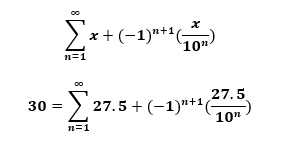This fancy looking formula is a recurrent progression such that value 27.5 increases and decreases by an ever-smaller amount (27.5 + 2.75 – .275 + .0275 – .00275 + .000275…) until it eventual reaches 30.00000000000000000… In other words, oscillating reflections of itself.

Moreover, 30 is the numerical value of Lamed(ל), the one letter associated with 42 through the 42 repetitions of the name Yehuda (יהודה) in the Torah and its complete value, which is 42. It is also the one letter associated with the level of Binah, as it is the only letter designed to reach upwards.

And since 3 is essentially 30/10, we also have: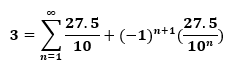Thus, we see that the 210-cubit height of the pyramid, or 5775” is purposely designed to be 3” shy of 5778 in order to leave room for higher plane or Binah hyperspace cubit to complete it.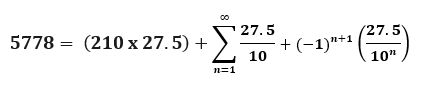### Phi and the Tower of Truth

And just to remind you, this applies to the Tower of Truth as well, which as we recall  it structured based on the sum of the cubes from 13203, meaning as recently explained, it is operating on a higher level.  And speaking of otherworldly connections, we have the 18 digit repeat sequence found in the Phi(φ) based Fibonacci numbers derived by dividing every 4th Fibonacci number by 3 sequentially in mod 9: 173557319826446289. Astonishingly, the virtual 21st cube in the Tower of Truth, associated with Binah through the Name Ehyeh (אהיה) yields the equation whereby the 21st cube’s volume, which is exactly 21% of the 44,100 cubits3 Tower of Truth’s 20 cubes, divided by the total volume of all 21 stacked Cubes or 9261/(441,000 + 9261) cubits3 = exactly .173553719826446289.

The connection here obviously originates in the dimension of Binah. But why the swirling confluence with Phi(φ), the Tower of Truth, and 18 once again?

### The Essential Cube of Creation, the Torah, and the 42-Letter Name.

How about the Torah, whose first verse totals 2701?

What is 1/27? It works out to .370370370370…, which is .3701 + .0002701 + .0000002701 + .0000000002701… Yet another equation yielding an infinite reflective sum, this time combining two Essential Elements. The value of the 42-Letter Name in our world (Malchut) is 3701 and that of the Torah’s first verse, which permutes into the 42-Letter Name is 2701. Their difference, 1000, indicates they are together in the realm of Binah hyperspace, prior to their separation to create our existence. Now we see how they are connected and where, all through the Essential Cube of Creation.

The small gematria of the 42-Letter Name in the level of Zeir Anpin is 173 or (20027), representing what the Zohar describes as the 173 keys to Heaven given to Moses. Connecting the numbers through the dimensional array we see that 1/5778 = .0017307026… and 1/173 = .005780

Notice that the (200 27) connects with the (200 x 27) equation that represented the total for the Magic Cube equation of 5400. The connections/interconnections are more important than the numbers themselves. You could have a cell number, but if there were not a satellite or a server to funnel that number through, what good would it do you? Here we see another element of the mathematics of the higher dimensions: the results of applying any of the operators (+, -, X, /) between the numbers are related. In this case 173 is related to 5400.

In the level of Binah, the 42-Letter Name is 1/27.  The Magic Cube of 42 is 27. Together they are 27 x 1/27 = One(1). “He and his Name are One.”

The number 378, the value of “Echad Ushmo Echad (אחד־ושמו־אחד)” or “His Name is One,” is (9 x 42).  The value 378, as the sum of the ordinal values, exists on the level of Zeir Anpin, and translates to 27 on the level of Binah. If on the level of Binah, the 42-Letter Name is 1/27 then on the level of Binah: Echad Ushmo Echad (אחד־ושמו־אחד)/42 = 27/27 = One(1).

The number 378, the value of “Echad Ushmo Echad (אחד־ושמו־אחד)” or “His Name is One,” is (9 x 42). When the level of Zeir Anpin is combined with the level of Binah: Echad Ushmo Echad (אחד־ושמו־אחד)/27 = (9 x 42)/27 = (1/3 x 42) = 14, the central cube.

### The Power of 9

The number 378, the value of “Echad Ushmo Echad (אחד־ושמו־אחד)” or “His Name is One,” is (9 x 42). On the level of Zeir Anpin: Echad Ushmo Echad (אחד־ושמו־אחד)/42 = (9 x 42)/42 = 9.

Twenty years ago, when I was first learning the secrets of the 42-Letter Name, Rabbi Akiva, of blessed memory, told me the true secret was in the power of nine(9). I soon learned that nine(9) divided by the small gematria of the 42-Letters was 9/.1230490… = 73.141593… or 70 plus Pi(3.141593) with a precision accurate enough to get man to Mars, and it is also conveniently a reference to the 9th sefira, Chochma, of numerical value 73, but the secret was much deeper.

The two Echads(אחד) for One in “Echad Ushmo Echad (אחד־ושמו־אחד)” total 26, as in G-d’s Name YHVH (יהוה), which operates at the level of Zeir Anpin, One(1) less than 27, which is at the level of Binah.  As stated above, 378 is 27 in the realm of Binah, and 27 is (10073). The more you know, the more you grow.

The negative space within the Magical Cube of 42, collectively is 22,005. As we saw, the natural logarithm of 22,005, or LN (22005) or Loge (22005), is 9.9990… or precisely 1/1000 less than a prefect 10, which exists in the level of Chochma, the 9th sefira (dimension), hence we cannot even see it. It has no physical attributes.  Another way of observing the above equation is e9.9990 = 22,005 with Euler’s number (e) being equal to 2.718281828459045…. It too starts off with the equation 27 = One(1). We see across all borders the interconnectivity of numbers, both irrational and rational, both illogical and logical, both natural and impossible.

Even the numbers in the positive and complimentary negative space sum to 9. The total 27,000 value reduces to (2 + 7) = 9. The components (22,005 and 4995) reduce to (2 + 2 + 5) = 9 and (4 + 9 + 9 + 5) = 27 = (3 x 9). The total 27,000/9 is obviously 3000, but the negative space portion (22,005/9) = 2445, the year Aaron turned 80, 3 years before the Exodus in 2448 HC and perhaps when the 10 plagues began. Moses was 77 at the time, which was 3333 years before 5778. The positive space (4995/9) is thus 555, associated with the Shechinah. The ratio of 2445/555 = 4.40541 with 44 being the numerical value of the 1st plague and the 541, Israel, being the value of all the initials of the 10 plagues. We have been looking at numbers in this chapter as portals to the higher dimensions, but are numbers passive descriptors or are they active operators influencing time, placement and action?

### Cycles of 7

Each face of the Magic Cube of 42 has a value of 126. The 3 central axes have a value of 126 collectively.  That is 7 sets of 126 that structurally create the Cube, as in the counting of time given to us in Genesis. The 6 surface days and 1 deeper day, split in 3 parts, that holds it all together, analogous to the 3 meals of Shabbat. Shabbat is the counter, the marker of time as we know it.  Each Shabbat marks a cycle of 7 days, one week. Counting from the Creation of Adam, there are (5779 x 52) = 300508 cycles of 7.  That is 358, Moshiach, once the dots are connected.

At 5780 cycles of 52 annual cycles we have 300560 total cycles of 7, which is numerically 4245 less then the 304,805 letters in the Torah.  For those that think that maybe the Creator did not know about Man’s counting of solar or sideral years, 5778 sideral years (365.256 days) is 301492 weeks (cycles of 7), as in the year 1492 when Columbus discovered America, whether revisionist hoodlums tear down his statues or not. The difference between counting cycles of 7 for 52 weeks every year for 5780 years and counting sideral days for 5780 years, is 20 years. In other words, 2020 CE vs 2000 CE.

### The Special Property of 9

Each of the 9 planes of the Cube, 3 along each of the 3 axes, have a collective value of 126.  The value 126 is (9 x 14), anchoring them all in the central cube of 14.  Is it a higher dimension design element of the number 5778 that the central two digits sum to 14?

And in thanks to the special property of 9, whereby the digits in any number divisible by 9 sum to 9: (1 + 2 + 6) = 9, and (3 + 7 + 8) = 18, and (7 + 5 + 6) = 18, and (5 + 4) = 9, and (2 + 7) = 9, and (5 + 7 +7 + 8) = 27. But what gave 9 its special property?

We are at the cusp of understanding numbers.  We are at the birth of enlightenment.

The numbers, the letters, the forces are One. The source is One.

And why is the bottom diagonal of the Cube (92718)? Look how much Tesla accomplished.

# Arrays and Expanding Space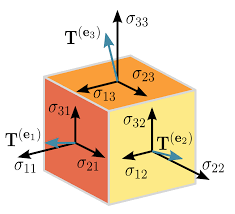We think of the 33 Cube of 27 numbered sub-cubes as static, but mathematicians know that each one of the 3 directions of the cube can be stretched or “stressed” in 3 directions, making 9 in total, along the 9 planes of the Cube.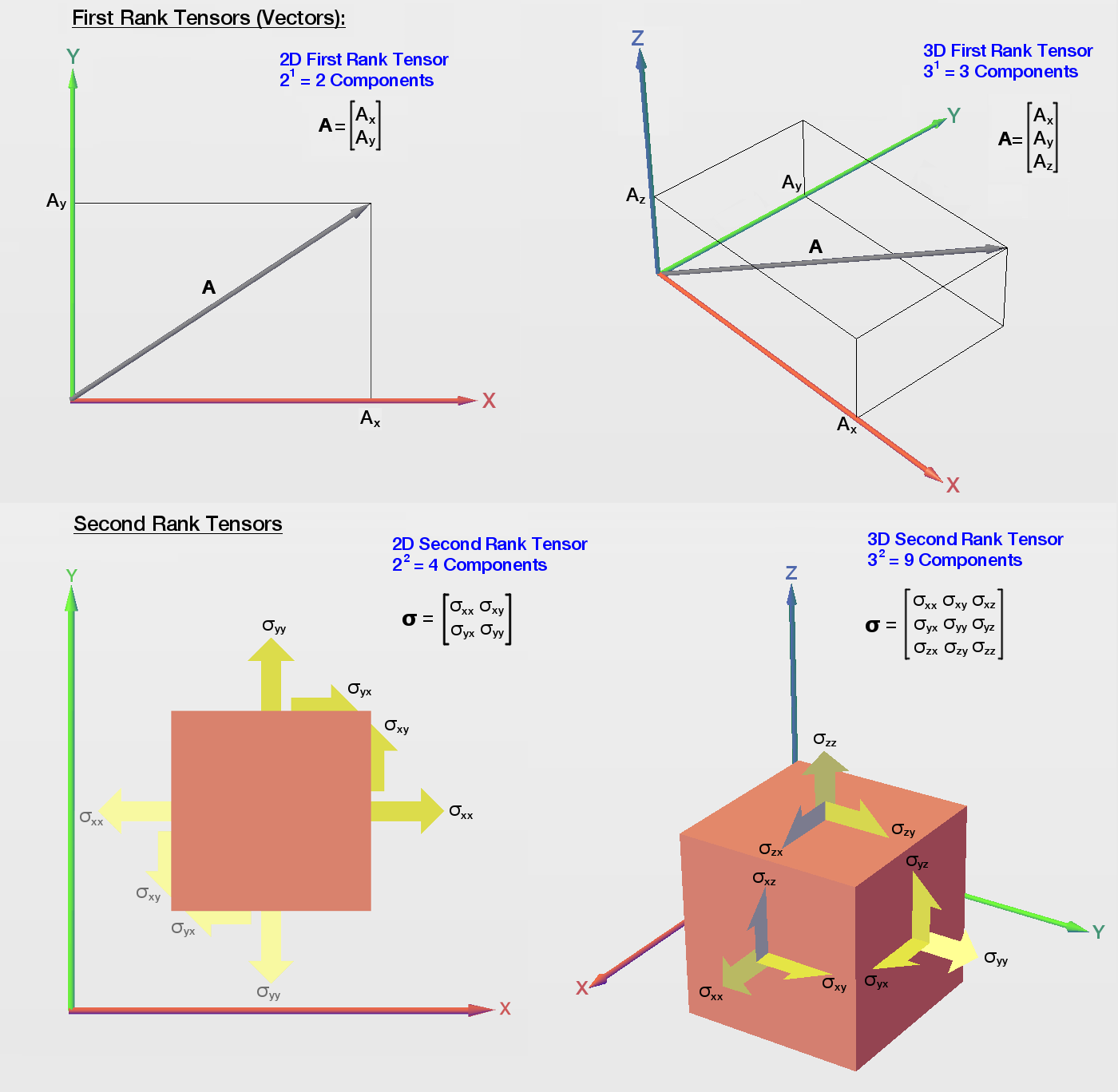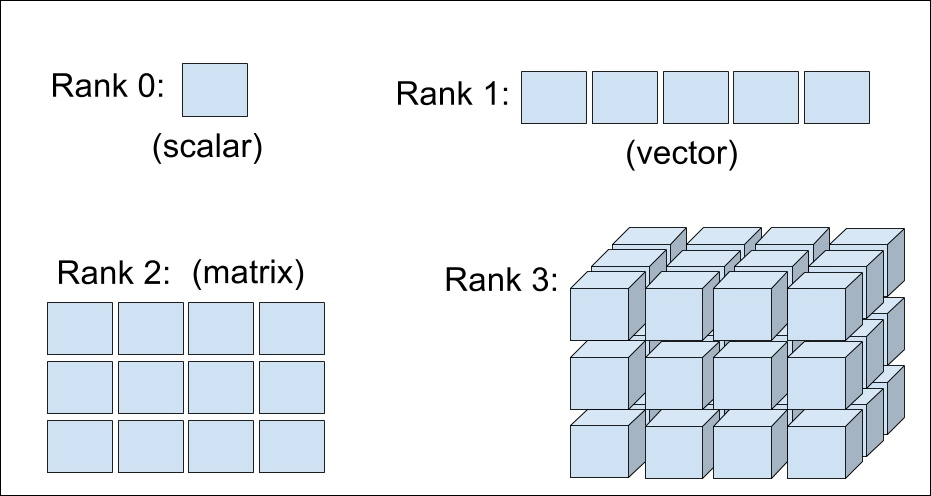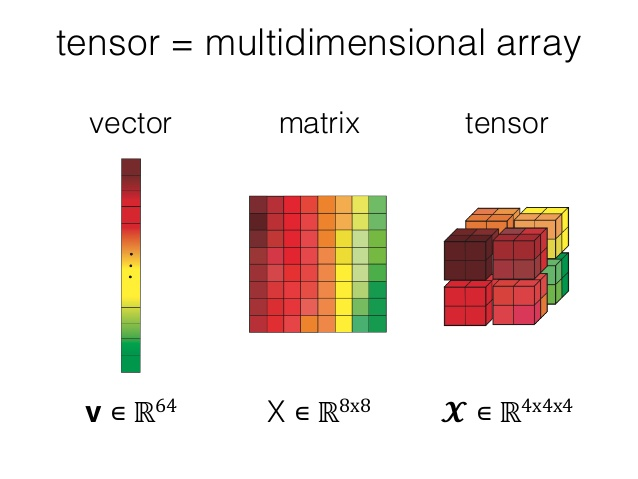This 33 cube is called a stress tensor, a Rank 3, 3 Dimensional stress tensor, also called a multi-dimensional array.

Rank 1 is a vector; Rank 2 is a matrix; and Rank 3 is an array. Each one of the sub-cubes has a coordinate placement (xyz) for each of the 3 axes from 1,1,1 to 3,3,3. If we add all 27 coordinates (111, 112, 121, 211…333) we get 5994, and (59944995) =  999. If we did not know about the interconnectivity of numbers, we would say so what. If we did not know that the 27 Letters in the Magic Cube of 42 was a Rank 3 Dimension 3 stress tensor array that summed to 4995, and that 4995 = (5 x 999), or that 27 = (9 + 9 + 9), or that natural logarithm of the negative space in that cube, Loge (22005), is 9.9990…, we might say so what. If we did not realize that 5994 = (6 x 999), as in the 6 directions and 6 bundled dimensions of Zeir Anpin, or that within the first 1000 digits of Pi there exists a numerical string of 6 9’s, …999999…, and that the 6 9’s sum to 54 we might say so what. If we did not further realize that that string of 6 9’s“…999999…” means that the numerical string …9999… appears 3 times in those 1000 digits, matching up with the other two triplicate 4-digit strings, …5778… and …2019… we might have said so what? If we had not realized that the sequence …999999… was part of a larger sequence in Pi, …999999837297804995…  and that 729 equals (9 x 9 x 9) or 272 and that it is the value of the 2nd line of the 42-Letter Name Matrix, we may have said so what? If we had not seen that this 18-digit sequence …999999837297804995…  connects the positive space (4995) with the negative space of the Magical Cube of 42 through Loge (22005), which is 9.9990, we may have dismissed it. The same goes for noticing that the sum of the digits within the 18 digit sequence through the number 72 is 72. It goes the same for the 6 9’s, …999999… that sum to 54, and the numerical string …4995… that sums to 27, effectively bookending the sequence with the numbers that define the Magical Cube of 42 on the level of Binah.  And let us not forget the connection between the 72 Triplets and the number of their collective square roots, 1000.054.

Since we did not miss any of it and since we know about the significance of connections between the numbers, we can understand one of the cosmic significances of our findings. When due to the counting methodologies previously discussed, the 2-year shift in the Hebrew calendar is taken into account and the 1-year shift in the Gregorian one is, we find that this 18 digit sequence is pointing us to the fact that the Magical Cube of 42, the Essential Cube of Creation, would be revealed in the year 5780 HC (999999837297804995) which is 2020 CE.

Since the triplicated 4-digit sequences of 9999 connects with 5778 and 2019 at the level of Binah the dating aspect in the 18-digit sequence is further emphasized with the numerical string …5778… found at digit #954, part of the fuller sequence …57781857780….

As mentioned in The Divine Calendar, the sum value of all the 947 digits in Pi up to the …57781857780… sequence is 4240, the value of Moshiach Ben David x 10.

And guess what number is found at digit #995, as in 4995? It is 4, and it begins the final sequence …420198. Moreover, working backwards from the start of the final sequence …420198 at digit #995 to the exact 4240, Moshiach Ben David summery point where we found …57781857780…, the sum of all those 48 digits is 210, as in the height of the Pyramid in cubits and levels and in its 48 stories. It is also the 210-year length of the Egyptian exile in years.  Without connections numbers are just numbers, pixels painting the limitations of our world.  We need to see the connections to begin to grasp their influence on our existence.

We began our discussion in this chapter with the values of Abraham and Aaron, 248 and 256 respectively.  We asked why these numbers were back to back in the Torah and in natural logarithms. Since Abraham died in 2123 HC and Aaron was born in 2365 HC there is a difference of 242 years between their lives, matching the 242 years between the birth of America in 1776 CE and 2018 CE (5778 HC). The Hebrew date for this momentous event was the 17th of Tammuz, the 4th month. The Western date was of course the 4th of July, 1776, matching the sequence in Pi at digit number 888, …171776…, which is additionally notable since 1776 is (2 x 888). Nevertheless, since Abraham was born in 1948 HC and Aaron was born in 2365 HC there is a difference of 417 years between their births, matching the 17th of Tammuz, the 4th month, which is also the date the Second Holy Temple’s walls were breached in 70 CE.  Twenty-one (21) days later the Holy Temple itself would fall.

### Gog V’Magog

Why is Gog V’Magog of numerical value 70 happening now in 2020? Can it be the connection to the two Shins (שש), of ordinal value 42, which when spelled out Shin-Shin (שין־שין) have a numerical value of 2020. Each of the Shins (ש) individually has the ordinal value of 21, as in the Name Ehyeh (אהיה) associated with Binah. The two Shins (שש) would thus be associated with the magical powers in the Name “I Am That I Am (אהיה־אשר־אהיה),” given to the Israelites in their darkest need in Egypt, as numerically it is (21 – 501 – 21) with an ordinal value (214221).  Life is all about timing. We understand that. It is more important to be at the right place at the right time or make the right investment at the right moment than being excessively smart or skilled.  Being in the right place at the right time also means not being in the wrong place at the wrong time which can be devasting even if you have everything else in the world going for you. But what if numbers are not passive? What if they are active participants in our world? What if they create pressure mediations in our world, lives, timelines?

If you recall from much earlier in this series, there are four (4) forms of energy movement in our world. These are influenced by the 27 higher dimensions pressure mediations and the fields they interact with. These are four (4) forms, directly analogous to the YHVH (יהוה):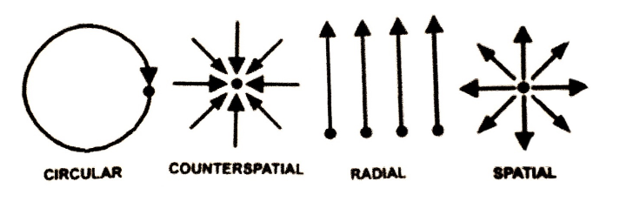Now, let us flip their order, as if we were reading from right to left, as in Hebrew. Again, the order makes no difference as far, as we know: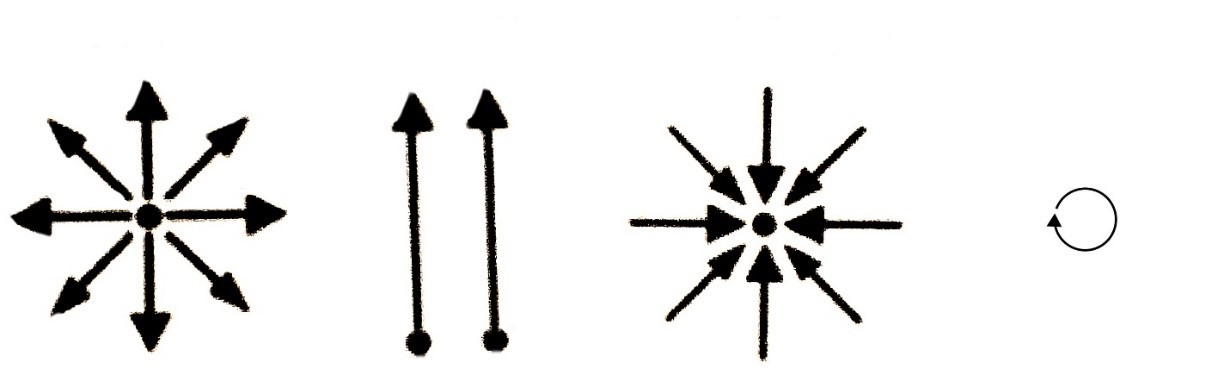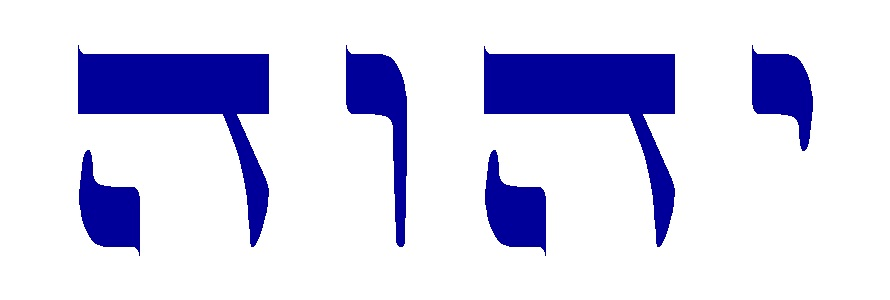Here is another View of a Stress Tensor, to give you an idea what these energy movements look like in 3-d, our world as we perceive it: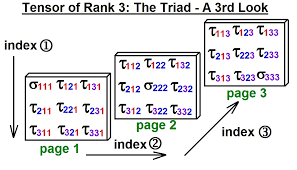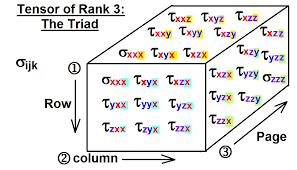Here is the Stress Tensor, Rank 3, Dimension 3, depicting one of the pressure mediations, the Spatial one.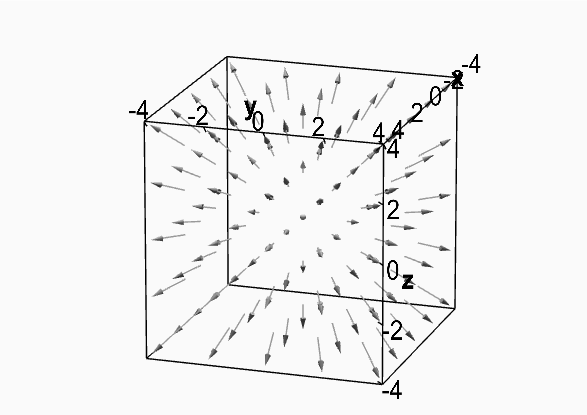What if the same number could have different pressure mediations depending on what was happening in the upper dimensions?  Like a magnetic that we see as repelling or attracting, depending.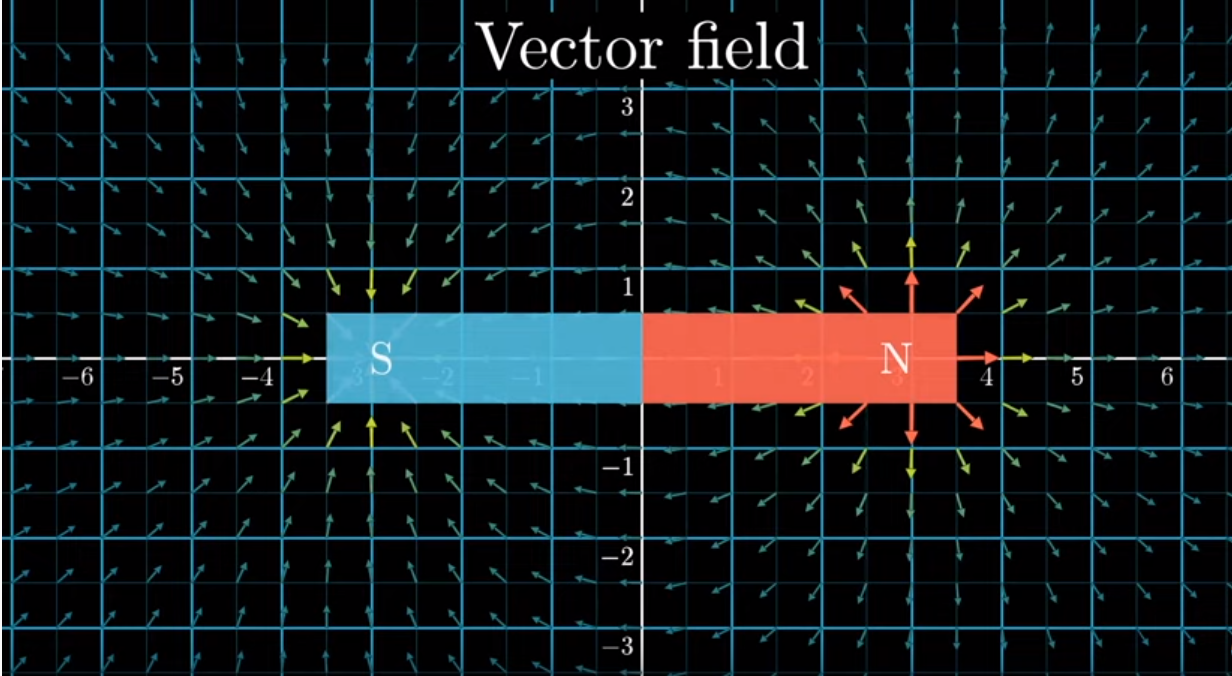What if the same number could have different pressure mediations depending on who we are, or more accurately, our consciousness. A soul is a soul and not of this world, but a consciousness that wraps around that soul like the negative space that defines the positive space can be good or evil or usually an admixture of it. What if the same number could have different pressure mediations depending on our consciousness, attracting some, repelling others, actively sorting us out based on our intentions, spoken or otherwise.  What if that number were a year or date on a timeline, sorting society into two camps?

How does a number decide? It does not. We do. Its qualities are programmed from the higher dimensions.  In our world, likes attract, not opposites, as you have been told. Good attracts good, evil to evil, order to order, chaos to chaos.  We see it with prominent people. Half the people hate Trump, half love him in spite of what 97% of the media and the Tech Titans tell them to think. It is inside us, or more specifically, wrapped around our soul. He has a number. Same with Obama.

### Euler and the Sea of BinahTo most of us, seeing the first 16 digits Euler’s Number, 2.718281828459045… we immediately realize that something mechanical must be going on with these digits (27, 18, 28, 18, 28, 45, 90, 45), especially since we know the constants originate in the sea of Binah, as I once discussed with Rav Brandwein.  That it begins with 27 must relate it to the Essential Cube of Creation, itself situated in Binah. Then 18, which keeps coming up, even in the mysterious Phi(φ) sequence associated with 21 and Binah, .173553719826446289, whose 18 repeating digits sum to 90. Some look at 2.718 and say it is weird that it is only .1 off of an abbreviated Phi(φ), 1.6180. Others say it is weird that (2 + 7 + 1 + 8) = 18 as in (27, 18, 28, 18, 28, 45, 90, 45) and (2 + 7 + 1 + 8 + 2 + 8) = 28 as in (27, 18, 28, 18, 28, 45, 90, 45), or that the pairs all sum as (9, 9, 10, 9, 10, 9, 9, 9) or 6 9’s (999999). Where have we seen that before?

As we begin to learn the connections behind all things, we see that (Pi – e) or (3.1415926… – 2.7182818…) = .423310… If you recall, the Adam and Eve ratio broke down to 19/45 or 42.22% and 57.78%, and the Tetragrammaton (יהוה) ratio of Chochma-Binah to Zeir Anpin-Malchut (וה to יה) broke down to 15 and 11, as in the 15 Triplets of the Shema and the 11 of Bereshit.  This gave us a ratio of 11/26 and 15/26 or .423 and .577 respectively. This seems to be highly reminiscent of the result above, .423310…, which was based on Euler’s Number, and also reminiscent of Euler’s Constant (.5772). Nevertheless, the result of (Pi – e) or .423310825… plus Euler’s Constant (.5772156649…) equals 1.00052649. Once again, we see the constants revealing themselves on the level of Binah (1000).

### The 11 Dimensions and Pi

If you missed the connection between the number 11, or the 11 dimensions of the sefirot including Da’at, and Pi and the 42-Letter Name you are forgiven.  Several times already we have illustrated the connection between the number 11 and aspects of the 42-Letter Name, including how the value of the first line (אבגיתץ) of the 42-Letter Name Matrix, and also its last 4 letters (וצית) have a value of 506, which is not only the complete value of Moshiach Ben David, but also the sum of the squares from 12112.  And we just illustrated how 9/.123049… = 73.141593… = 70 and Pi.  What we never explained before was that Pi in Base 2 or binary, the language of our computers, is 11.001… and the sum value of the 42-Letter Name, 3701/11.00100 = 336.424 or the 336 letters in the 112 Triplets and 424, Moshiach Ben David in standard gematria.  As for the 22,005 units of negative or complimentary space, 22005/11.001001 = 2000.273, with the digital string …2000… also found in Pi at digit #601. The mathematics of at least 3 different dimensions are at play here at once.

The question is not How, it is Why.  We cannot fathom “How,” but pondering “Why” leads to elevation.

The more you know, the more your consciousness expands. As previously explained, Pi is infinite, circles are infinite, and the Essential Cube of Creation expands (stresses) in 9 directions. There is no reason our consciousness cannot likewise expand. The more our consciousness shifts towards “good and order” the more the right numbers will be attracted to us and us them to further our expansion. Why order? Remember, there is nothing random in the universe. We can chose to see the order and the existence of the Creator in all things, or our consciousness can choose to see chaos like we have in our streets today. This is the meaning of the War of Gog U’Magog.

The most important connections are found in the least likely places.  Numbers are not what we think they are.

We said previously that each of the 9 planes of the Cube, 3 along each of the 3 axes, have a collective value of 126.  There are two more less obvious planes in the Essential Cube of Creation, the diagonal planes across the Cube, making 11 planes in total, each with a collective value of 126, or since each one is 14(101), collectively they are 14 (1001) in total. Why does each plane of the Essential Cube of Creation have to be (3 x 42), and also 14(101)? Do no ask How, ask Why. Why is it important that 9 be (101)?

### A Question about a Prophecy

Most of us living today, could not miss the marker in Pi and in the equation above (2000) and associate it with time, as in the year 2000 CE. As for 273 in the result 2000.273 we recall that it is a number at the center of the dimensions of our solar system and the temperature of our universe and that it squares the circle, but in higher dimensional mathematics it is also 273 and (30027). Moreover, (2 x 7 x 3) = 42, giving it a P/S ratio of 42/12 or 3.5, also known as “Time times and half a time.” (Daniel 7:25 and Daniel 12:7) Why, not How.

### A Question About the Pyramid

Since the base of the Pyramid, 756’ equals the 6 faces of the Cube (6 x 126 = 756), we should also ask why (7 X 5 x 6) = 210, the height of the Pyramid in cubits, or that the a P/S ratio of the Pyramid’s height (481.25’) is 320/20 = 16, or why together (320/20 and 210/18) their P/S ratios are (16 + 11.666) = 27.666, exactly 1/6th more than the cubit itself 27.5”?

Did 18 popping up again catch your attention, or did the denominators being 20 and 18, or did the 16 as in the 16 digits of Euler’s Number? Some pseudo-scientists will tell you that you only find what you focus on and that that is why you can make connections between numbers that others take for granted.  What if the numbers are instead seeking you out? Or more accurately, the higher dimensional forces behind the numbers. Nothing happens in a vacuum. In fact, there is no nothing.

# A Secret to Redemption and the 42 Letter Name

Remember that special 18-digit sequence within Pi …999999837297804995… , well the digits in it that we had not highlighted (8378), not only include 378, but are also a value of 22 less than 8400, or (840022).  Connections are everything. The gematria translation of 84 is Pad, redemption, and as Rav Brandwein, of blessed memory, explained to me, it is the fusion of the Upper and Lower 42-Letter Names. As it so happens the natural log of 8400, or Loge (8400), is 9.0359, and on average, each of the 42 rows in the Torah, spanned across all 248 columns has a combined average total of 9300.59 words, letters, and verses. Keep in mind that the complete (Zeir-Anpin-Malchut) value of ruach (רוח) that permeates the Essential Cube of 42 is 248. The Spirit of G-d spans across all 248 columns of the Torah Matrix. Meanwhile, 273223 = 9035.  First there was the array, then there was the Essential Cube of Creation, then there was Matrix and then came the Torah to fill it.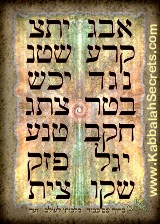To operate in hyperspace and break this down a little further, the number 8400 is (42 x 200), as in the equations (20027) and (200 x 27) that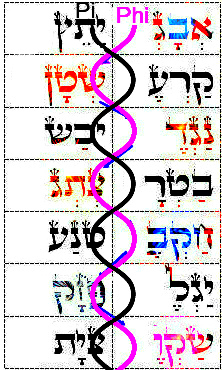we just saw above with the 42-Letter Name and the Magical Cube of 42 respectively. Moreover, the number 903 is the sum of all the integers from 1 – 42, higher dimensional math at work.   We also saw 903 in Bereshit (בראשית) when broken down to Beresht (בראשת) and Yud (י), or 903 and 10; and again earlier as 903 times the square root of 5, which is the core of Phi(φ), that equals 2019.17. Even earlier we realized that when using the same zig-zag method of climbing the 14 Triplets in the 42-Letter Name that revealed Pi and Phi(φ), we found the 2 column in the 11 Triplets of Bereshit total 903 and 1959 respectively, giving us 90359 as an extraction. The even much earlier, we saw 903 again as in 9 of the 10 Utterances in Bereshit that all begin with “G-d said, Vayomer Elohim” whose 10 Hebrew letters (ויאמר־אלהים) total 903.  We now know higher dimensional math also reveals that so apropos simple equation that (9 x 3) = 27, and 93 = 729, the second line of the 42-Letter Name Matrix, while the square of its P/S (27/12) is 5.0625, the first line of the 42-Letter Name Matrix and H’Keter (625), the square root of all the words, letters and verses in the Torah.

We can no longer look at numbers linearly.

We can now understand that the number 14 at the center of the Magical Cube of 42 has a deep connection to the 14 Triplets in the 42 Letter Name; as is the 3.14 in Pi; and the Archangel named Metatron of gematria value 314; and the Name of G-d Shaddai (שדי).

### I Am That I Am (אהיה־אשר־אהיה)

If we take all 3 levels at once, the value 1 for Alef(א) becomes (1 + 1 + 1); 2 for Bet (ב) becomes (2 + 2 + 2); 10 for Yud(י) becomes (10 + 10 + 10) = 30; 11 for Caf(כ) becomes (11 + 20 + 11) = 42, making Caf-Yud(כי) equivalent to 72; then the central 14 for Nun (נ) becomes (14 + 50 + 14) = 78 or (3 x 26). Using higher dimensional mathematics again, reveals that the total of the 27 square roots of all 27 letter-number combinations is 314.278.  This is readily reminiscent of Pi, Metatron, and the Name of G-d, Shaddai (שדי), all of numerical value 314, and it is coupled with 278, the numerical value of “The gematria.” It is further reminiscent of 3142, referencing the name “I Am That I Am (אהיה־אשר־אהיה),” at Exodus 3:14. We also saw 3142 in the simple equation dividing the total value of the 42-Letter Name matrix by 506, its first line, which is also the complete value of Moshiach Ben David, or 3701/506 = 7.31422. We now understand that when utilizing the complete value of a letter, word, phrase, verse, etc. we are observing the combined or connected force of Zeir Anpin with Malchut. For example, while the ordinal (Zeir Anpin level) value of Asher (אשר) is 42, the complete combined (Zeir AnpinMalchut) value of each of the higher Names Ehyeh (אהיה) is also 42.

Here is a key to magical powers in the Name “I Am That I Am (אהיה־אשר־אהיה).” Beyond the triple 42, we see that the sum of the 3 levels (Binah-Zeir Anpin-Malchut) of the Name are (543 + 84 + 84) = 711, which is equal to the sum of all 22 ordinal values (711) of the entire Alef-bet spelled out, in other words, at full strength. This gives the Name “I Am That I Am (אהיה־אשר־אהיה)” mastery over the forces of the Alef-bet at the level (dimension) of Zeir Anpin, but only when all 3 levels of understanding are archived. And this is achieved through all 3 levels of the 42-Letter Names.

Could this be related to the primal equation where we took all the prime numbers (2, 3, 5, 7, 11, 13, 17, 19, 23… as a string of digits (.235711131719….) and then divided One (1) by them to get the Primal Equation 1/.23571113… = 4.24248100… or 42 and 4248, which is the sum of all 22 letters of the Alef-bet spelled out.  Thus, the Alef-bet’s ordinal value (711) and its gematria value (4248) are interconnected through the prime numbers.  Do we really have any understanding of numbers at all? Can we? Not if our consciousness remains on this limited plane.

Or the purpose of numbers? While we find asher (אשר) built into the first 4 letters of the Torah (בראש), we also find 3142 engraved, overlapping it, right into the first 7 letters of the Torah (ברא־שיתב) with small gematria (2213142). And using ordinal values, which we now know are on the level of Zeir Anpin, we have (221) built into the first 3 letters, illustrative of the 2 Names of value 21, Ehyeh (אהיה) in “I Am That I Am (אהיה־אשר־אהיה). The numerics, as we just saw with the primes and see repeatedly with Pi, whereby the numbers convey cryptographic messages, operate on the level of Binah. In the case of the ordinal values within Bereshit, these too give us (221). And the ordinal values within Bereshit (בר־אשית) can also break down sequentially to 22 and 54, while the total 76 is the natural log of 2000 or LN (2000) = 7.600…This gives whole new meaning to “In the Beginning G-d Created.”

There was G-d, then He Created the Beginning, then there was “In the beginning G-d created.” This changes the meaning to existence.

# אהיה

The real kabbalists knew that each level of expansion took them one level of understanding higher into the sefirot. They were looking at Zeir Anpin and then Binah with each expansion so we can now understand why the two most powerful Names, Ehyeh (אהיה) and the Tetragrammaton (יהוה), when expanded into 3 dimensions (iterations) together also equal 3142.

### אלף־למד־פא־הי־יוד־יוד־ויו־דלת־הי־יוד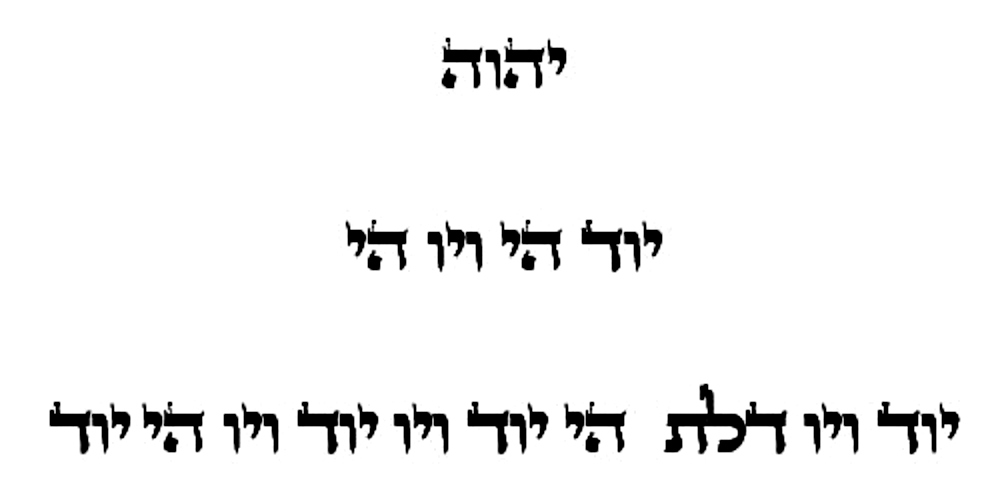The beginning was not so simple. So much went on before Elohim, the Torah’s 3rd word.

As explained once before in this series, the expanded components of Ehyeh (אהיה) are (506 + 110 + 1818) = 2434 and those of the Tetragrammaton (יהוה) are 708, as in the year 5708 when Israel became a nation. Together (2434 + 708) = 3142.  We now know about the Magical Cube of 42, and the importance of the central cube of 14, so is the equation (2434 + 14) = 2448, as in the date the Torah was given at Mt Sinai, a necessary part of the grand design?

If the expanded Name Ehyeh (אהיה) was conceived as deriving from Alef(א) at the level of Chochma, the 4 iterations, or 4 dimensional levels, would total 42 letters and the first 3 levels would total 903, as in all the integers from 1 – 42.  By the way, the final level has 27 letters and Alef(א) is One(1), so it expands from 1 to 27 to encompass 42, just like the Essential Cube of Creation.

### אלף־למד־פא־הי־יוד־יוד־ויו־דלת־הי־יוד

It only took 1 Alef(א) to create the 14 Triplets of the 42-Letter Name and it took 7, or (6 plus 1) to create the 11 Triplets of Bereshit. And it took 14 of them to create the 72 Triplets, and since not a one was used in the 15 Triplets of the Shema and Upper 42-Letter Name, 22 in total were used to create the 112 Triplets of Creation, corresponding to the 22 letters of the Alef-bet. While there are no Alefs(א) in the 15 Triplets that represent the Shema, there are exactly 42 words in the actual Shema that have the initial letter Alef (א).

One last parting gift, the complete (Zeir Anpin-Malchut) value of Moshiach(משיח) is 410, the same as Shema(שמע). When you recite the Shema, how many levels can you now consciously connect to? The same can be asked when you recite the 42-Letter Name? How do we elevate?

Like the 18 digits in the special sequence within Pi …999999837297804995… and the 18 in the …57781857780…sequence and in the equation Phi(φ)18 = 5778.000, there are a total of 18 planes within the Essential Cube (9 horizontal/vertical and 9 diagonal ones), each equal to 126 for a total of (54 x 42).

How much true beauty awaits us once the numbers that surround us are connected through the hierarchy of dimensions.

For anyone that has not been following this series from its inception, you can find the entire work here.